## 5.1Structure of matrices

As described in Sec. 3.4 , a matrix equation for solution variable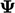contains of a set of coeﬃcients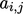where each rowcorresponds to the linear equation for the cell with indexas follows: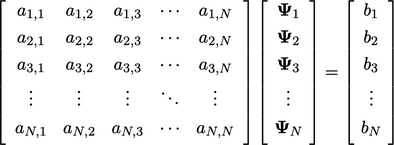(5.1)

### Matrix sparsity

Imagine creating a matrix equation for a transport equation for a scalar ﬁeld, e.g. Eq. (2.65 ) with zero heat source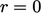.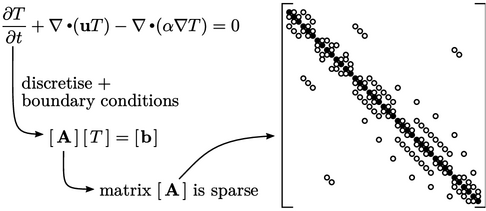The ﬁgure shows a matrix of size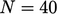, where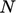is the number of cells. Circles denote non-zero coeﬃcients, which are ﬁlled for the diagonal coeﬃcients (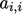). The matrix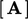is sparse, i.e. the majority of the coeﬃcients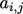are 0 (zero).

The sparsity is due to each cell interacting only with adjacent cells connected through its faces. For example, a 3D mesh of hexahedral cells produces up to 7 coeﬃcients per matrix row, with one diagonal coeﬃcient corresponding to a particular cell and 6 oﬀ-diagonal coeﬃcients for the neighbour cells.

### Matrix (a)symmetry

A symmetric matrix possesses the same coeﬃcients across the diagonal, i.e.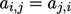. The discretisation of a Laplacian term, e.g.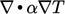, described in Sec. 3.7 , produces coeﬃcients that are symmetric because the surface normal gradient Eq. (3.5 ) uses the current and neighbour cell values in equal measure.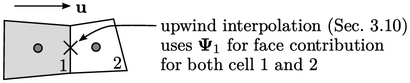However, the discretisation of an advection term, e.g.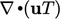, generally produces asymmetric coeﬃcients. For example, if the upwind scheme is applied for ﬂow from cell 1 into cell 2, then there would a contribution to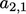but not to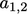.

### Matrix size

Parallel simulation allows aﬀordable solution on huge meshes. For a mesh of size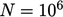, the matrix would have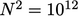coeﬃcients, typically with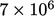that are are non-zero.

For reasons of eﬃciency, zero coeﬃcients are not stored in the computer’s memory. Instead the storage provides an array of non-zero coeﬃcients and addressing arrays of the corresponding row and column indices for each coeﬃcient.

Notes on CFD: General Principles - 5.1 Structure of matrices